# Chapter 5 Chapter 5: Introduction to Statistics in R

install.packages("ggplot2",  repos = "https://cran.us.r-project.org")
install.packages("dplyr",  repos = "https://cran.us.r-project.org")
install.packages("ggfortify",  repos = "https://cran.us.r-project.org")
library(ggplot2)
library(dplyr)
library(ggfortify)
lady <- read.csv("/Users/peteapicella/Documents/R_tutorials/GSwR/ladybirds_morph_colour.csv", header = TRUE)

View data structure:

str(lady)
## 'data.frame':    20 obs. of  4 variables:
##  $Habitat : chr "Rural" "Rural" "Rural" "Rural" ... ##$ Site        : chr  "R1" "R2" "R3" "R4" ...
##  $morph_colour: chr "black" "black" "black" "black" ... ##$ number      : int  10 3 4 7 6 15 18 9 12 16 ...

## 5.1$$\chi$$$$^{2}$$ contingency table analysis

Create new dataframe, totals:

totals <- lady %>% #working with this dataframe
group_by(Habitat, morph_colour) %>% #we want to represent these groups in the dataframe
summarise(total.number = sum(number)) #add up the numbers of each group using the new object total.number
## summarise() has grouped output by 'Habitat'. You can override using the
## .groups argument.
totals
## # A tibble: 4 × 3
## # Groups:   Habitat 
##   Habitat    morph_colour total.number
##   <chr>      <chr>               <int>
## 1 Industrial black                 115
## 2 Industrial red                    85
## 3 Rural      black                  30
## 4 Rural      red                    70

Create bar chart:

base_plot<-ggplot(totals, aes(x=Habitat, y=total.number,
fill = morph_colour)) + #fill is used when there is something like a bar that can be filled with color
#if this were color = morph_colour, then the argument would affect the bar's outline
geom_bar(
stat = 'identity', #this tells ggplot not to calculate anything from the data and just display the data as they are in the dataframe
position = 'dodge' #this is request to put the two bars in each Habitat group next to each other
#if it's not used, a stacked barplot would be printed
)
base_plot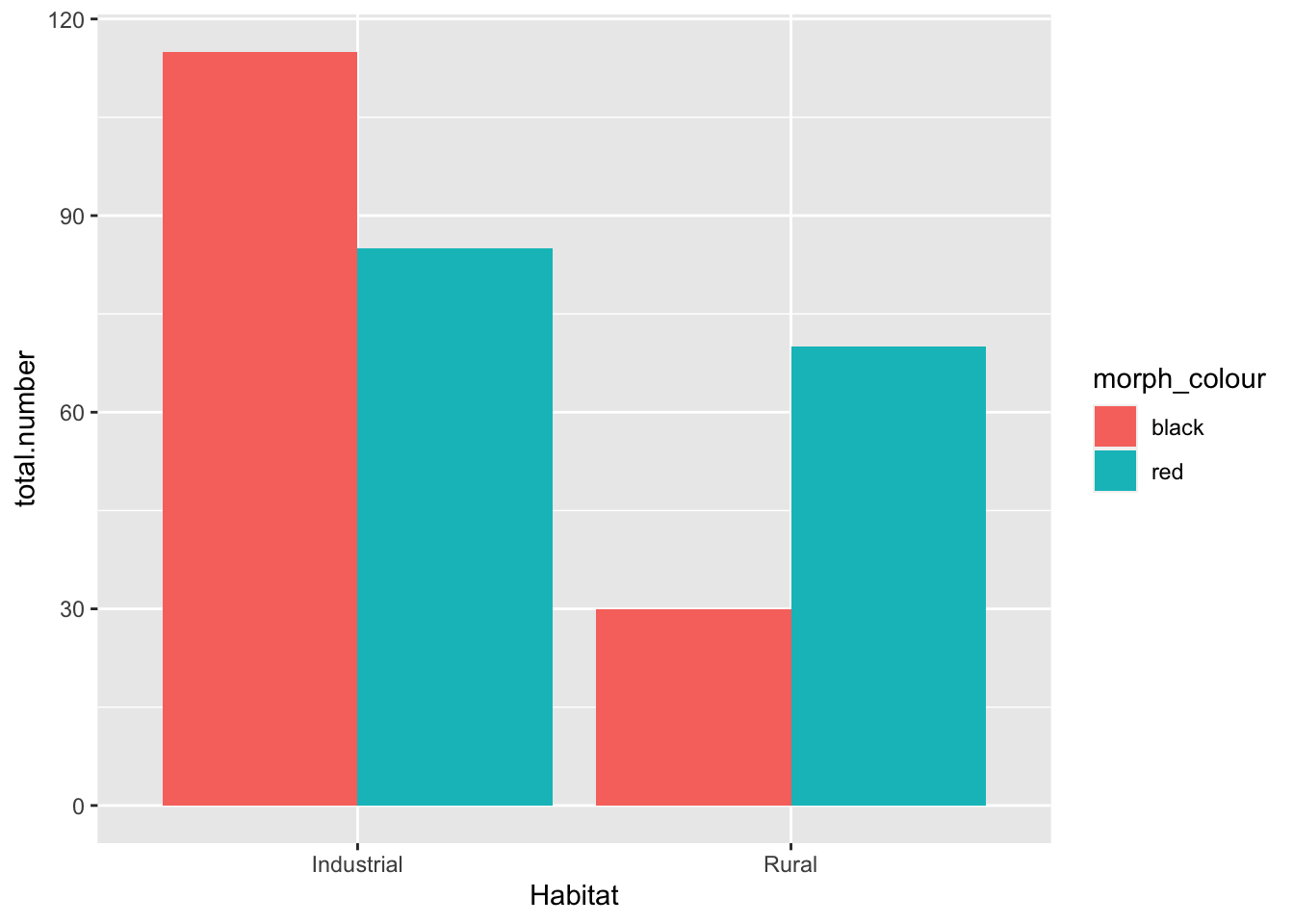base_plot +
scale_fill_manual(values = c(black = "black", red = "red")) #the text in "" are the colors we are instructing R to fill the bars with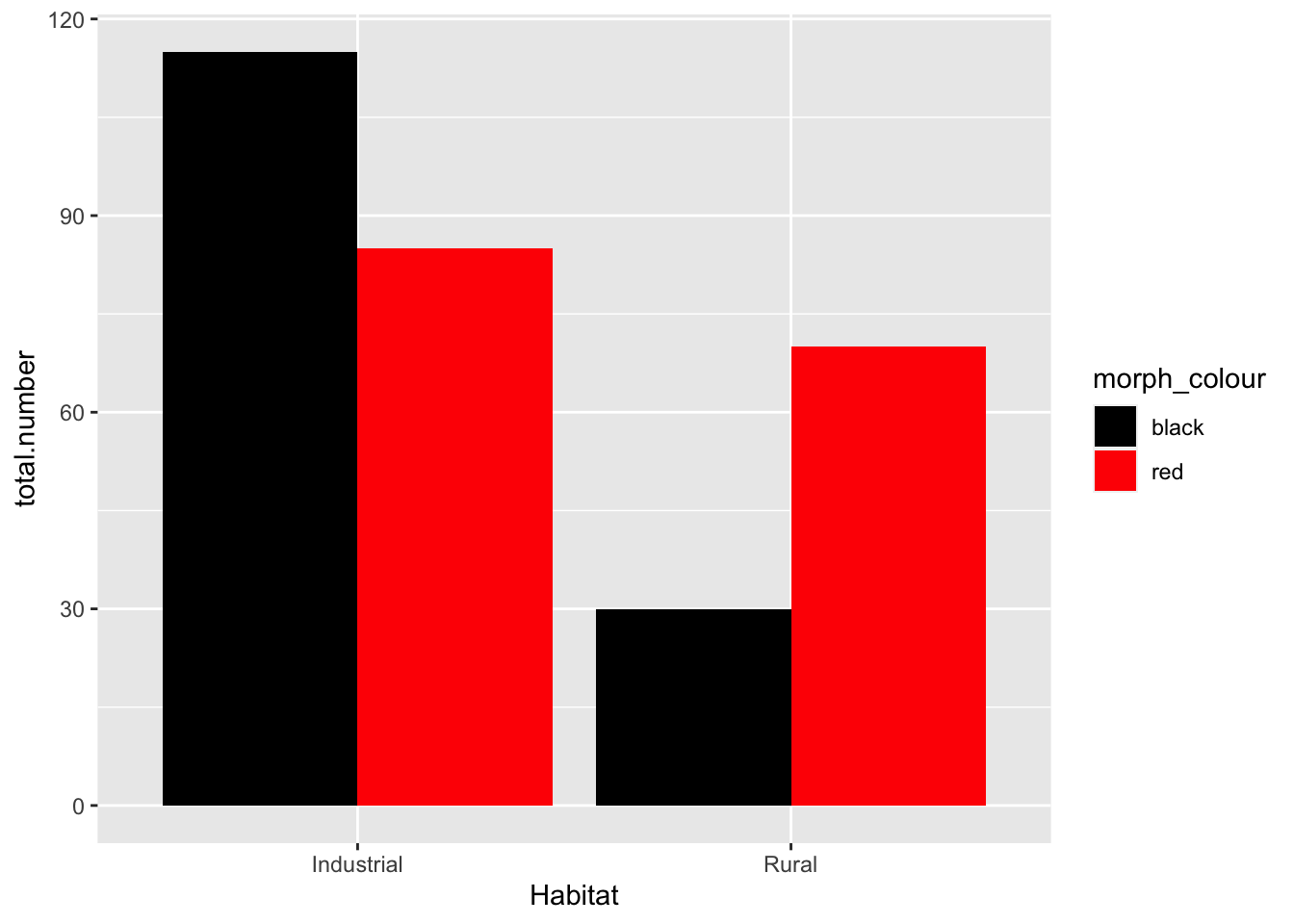• Null hypothesis: there is no association between the color of the birds and their habitat
• My opinion: data suggest that there is a higher proportion of colored birds in the industrial habitat. We should reject the null hypothesis (pre-stats)
• to rigorously test this, a chi-squared test must be performed

## 5.2 Making the $$\chi$$$$^{2}$$ Test

Use xtabs() function to generate a contingency table:

lady.mat <-xtabs(                     #function converts dataframe into a matrix, which is different from a dataframe
number ~ Habitat + morph_colour, #cross-tabulate the number of column counts in the dataframe by the Habitat and morph_colour variables
lady.mat
##             morph_colour
## Habitat      black red
##   Industrial   115  85
##   Rural         30  70

Perform a $$\chi$$$$^{2}$$ test on the matrix:

lady.chi<-chisq.test(lady.mat) #chi squared test function performed on matrix
lady.chi
##
##  Pearson's Chi-squared test with Yates' continuity correction
##
## X-squared = 19.103, df = 1, p-value = 1.239e-05
• the p value is 0.00001239 - this is the probability that the pattern arose by chance.
• It is lower than 0.05, so we can reject the null hypothesis
names(lady.chi) #can examine all of the parts of the test mechanics 
##  "statistic" "parameter" "p.value"   "method"    "data.name" "observed"
##  "expected"  "residuals" "stdres"

## 5.3 Two-sample t-test

• The two-sample t-test compares the means of two groups of numeric values
• It is appropriate when the sample sizes of these two groups is small
• The analysis makes the following assumptions about the data:
• The data are normally distributed
• The variances of the data are equivalent
ozone <- read.csv("/Users/peteapicella/Documents/R_tutorials/GSwR/ozone.csv")
glimpse(ozone)
## Rows: 20
## Columns: 3
## $Ozone <dbl> 61.7, 64.0, 72.4, 56.8, 52.4, 44.8, 70.4, 67.6, 68.8, … ##$ Garden.location <chr> "West", "West", "West", "West", "West", "West", "West"…
## $plant.growth.rate <dbl> 21.31695, 27.03072, 38.98937, 30.19529, 37.06547… ### 5.5.1 Getting and plotting the data Plot the plant growth (pg) data: ggplot(plant_gr, aes(x=soil.moisture.content, y=plant.growth.rate)) + geom_point()+ ylab("Plant Growth Rate (mm/week)") + theme_bw()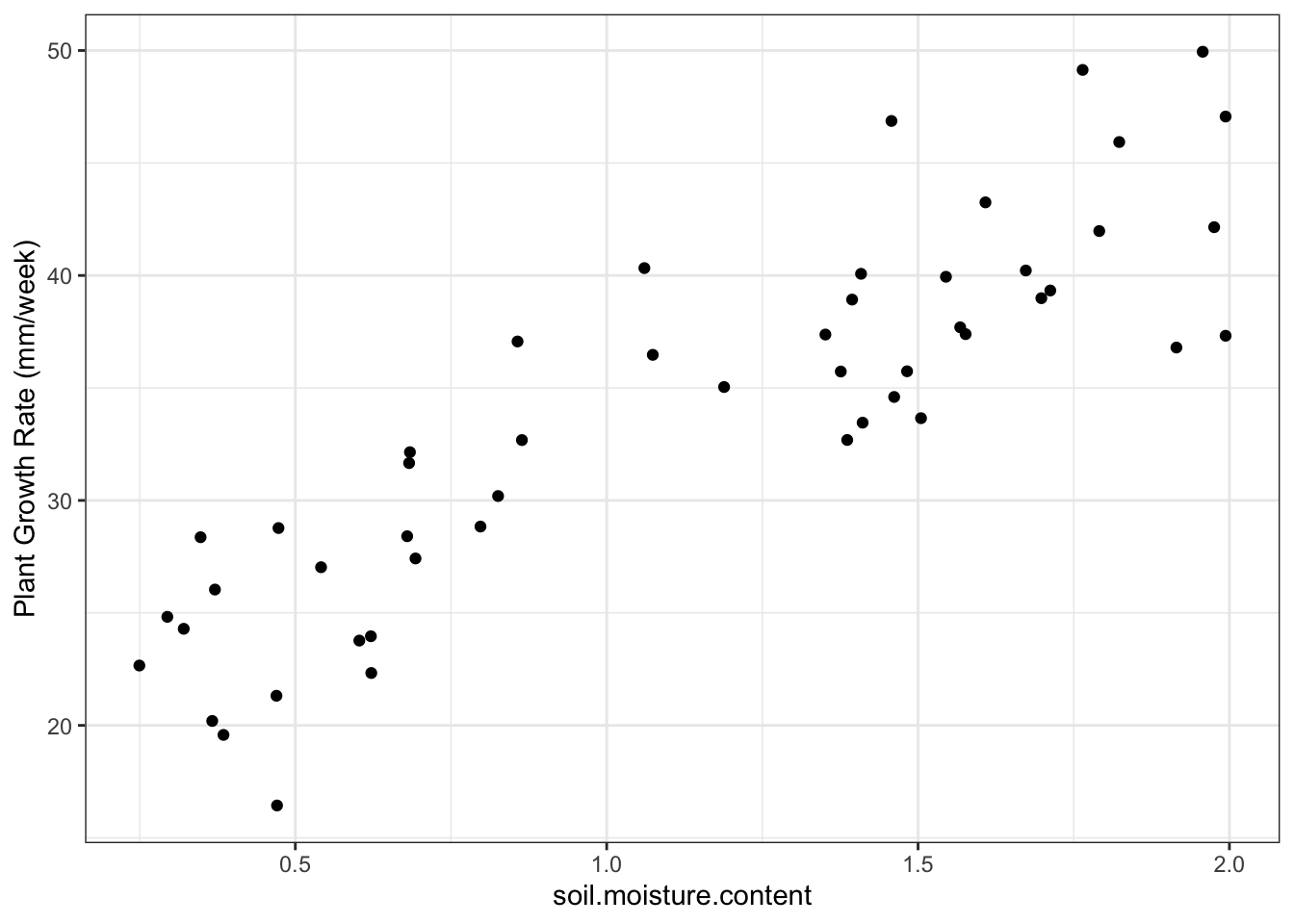• Plot shows that there is a probably a positive linear relationship between the two variables • There is a slope of about 15 mm/week • preliminary analysis: probably will reject the null hypothesis that soil moisture does not affect plant growth ### 5.5.2 Making a simple linear regression happen Create a linear model: model_pgr <- lm(plant.growth.rate ~ soil.moisture.content, data = plant_gr) #plant growth rate is a function of soil moisture content model_pgr ## ## Call: ## lm(formula = plant.growth.rate ~ soil.moisture.content, data = plant_gr) ## ## Coefficients: ## (Intercept) soil.moisture.content ## 19.35 12.75 ### 5.5.3 Assumptions first Check assumptions with ggfortify: autoplot(model_pgr, smooth.colour = NA)• ggfortify plots: • Top left - informs us on whether the line is an appropriate fit to the data; flat line means model is a good fit. Humps/valleys -> poor fit • Top right - dots = residuals; dashes line = expectation under normal distribution. Better tool than histogram to assess normal distribution • Bottom left - evaluates assumption of equal variance. linear models assume that variance is constant over all predicted values of the response variable. There should be no pattern • Bottom right - evaluates leverage. Used to detect influential datapoints that will shift the gradient more than expected + also for outliers • null hypothesis: soil moisture has no effect on plant growth ### 5.5.4 Now the interpretation Produce a sum of squares table: anova(model_pgr) ## Analysis of Variance Table ## ## Response: plant.growth.rate ## Df Sum Sq Mean Sq F value Pr(>F) ## soil.moisture.content 1 2521.15 2521.15 156.08 < 2.2e-16 *** ## Residuals 48 775.35 16.15 ## --- ## Signif. codes: 0 '***' 0.001 '**' 0.01 '*' 0.05 '.' 0.1 ' ' 1 • Output: • Large F value indicates that error variance is small relative to the variance attributed to the explanatory variable • This leads to the tiny p value. • Both are good indications that the effect seen in the data isn’t the result of chance Produce a summary table: summary(model_pgr)  ## ## Call: ## lm(formula = plant.growth.rate ~ soil.moisture.content, data = plant_gr) ## ## Residuals: ## Min 1Q Median 3Q Max ## -8.9089 -3.0747 0.2261 2.6567 8.9406 ## ## Coefficients: ## Estimate Std. Error t value Pr(>|t|) ## (Intercept) 19.348 1.283 15.08 <2e-16 *** ## soil.moisture.content 12.750 1.021 12.49 <2e-16 *** ## --- ## Signif. codes: 0 '***' 0.001 '**' 0.01 '*' 0.05 '.' 0.1 ' ' 1 ## ## Residual standard error: 4.019 on 48 degrees of freedom ## Multiple R-squared: 0.7648, Adjusted R-squared: 0.7599 ## F-statistic: 156.1 on 1 and 48 DF, p-value: < 2.2e-16 • produces table of estimates of the coefficients of the line that is the model • the slope that is associated with the explanatory variable (soil moisture) - the values of which are associated with the differences in plant growth rate Superimpose linear model onto plot: ggplot(plant_gr, aes(x=soil.moisture.content, y=plant.growth.rate)) + geom_point()+ geom_smooth(method = 'lm') + #put a linear-model fitted line and the standard error of the fit using flash transparent gray onto graph ylab("Plant Growth Rate (mm/week)") + theme_bw() ## geom_smooth() using formula 'y ~ x'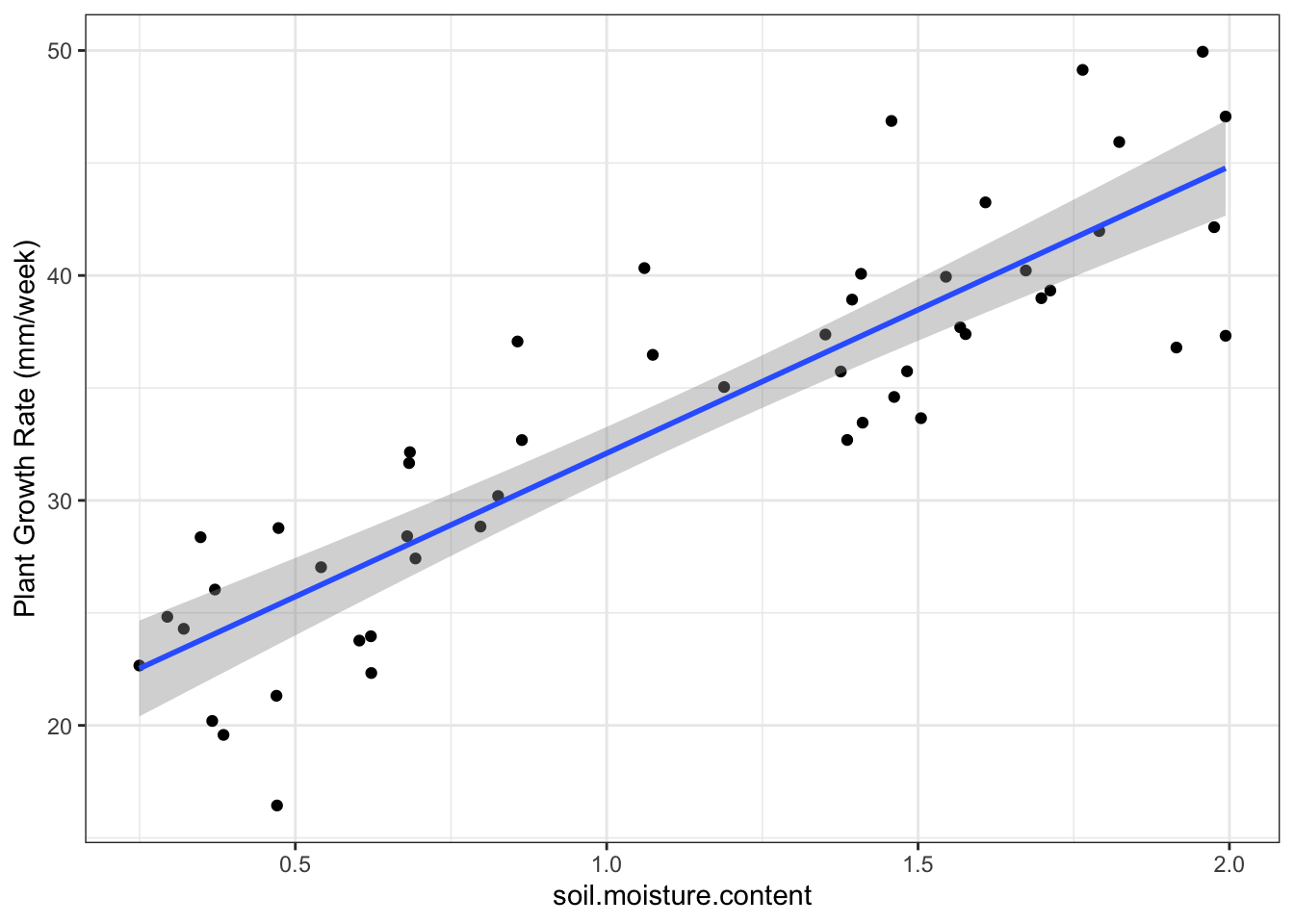## 5.6 Analysis of variance: the one-way ANOVA • the one-way ANOVA is similiar to the previous example and the two-sample t-test with one key difference: • the explanatory variable in the one-way ANOVA is a categorical variable (factor) • waterflea dataset - we ask: • whether parasites alter waterflea growth rates • whether each of three parasittes reduces growth rates relative to a control in which there was no parasite daphnia <- read.csv("/Users/peteapicella/Documents/R_tutorials/GSwR/Daphniagrowth.csv", header = TRUE) glimpse(daphnia) ## Rows: 40 ## Columns: 3 ##$ parasite    <chr> "control", "control", "control", "control", "control", "co…
## $rep <int> 1, 2, 3, 4, 5, 6, 7, 8, 9, 10, 1, 2, 3, 4, 5, 6, 7, 8, 9, … ##$ growth.rate <dbl> 1.0747092, 1.2659016, 1.3151563, 1.0757519, 1.1967619, 1.3…

Plot daphnia data:

ggplot(daphnia, aes(x = parasite, y=growth.rate)) +
geom_boxplot() +
theme_bw() +
coord_flip() #flips the orientation of the graph 90˚ to the right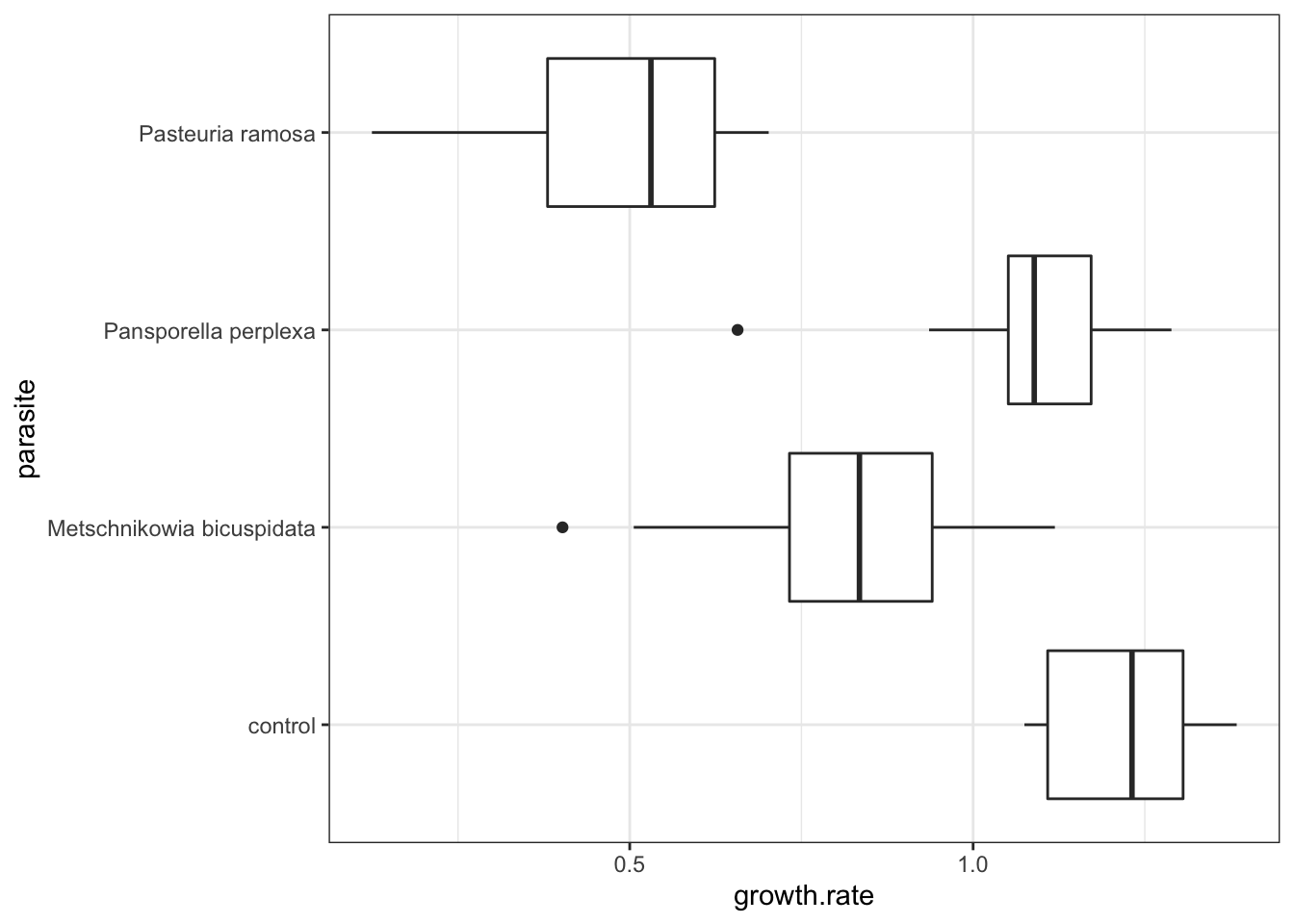• Visualization takeaways:
• There is substantial variation among the parasite groups
• The control group has the highest growth rate
• There is likely to be an overall effect of parasites on growth rate
• There may be an order in the impacts different parasites have on growth rates: P. ramosa < M. bicuspidata < P. perplexa

Create a model:

model_grow <- lm(growth.rate ~ parasite, data = daphnia)
model_grow
##
## Call:
## lm(formula = growth.rate ~ parasite, data = daphnia)
##
## Coefficients:
##                       (Intercept)  parasiteMetschnikowia bicuspidata
##                            1.2139                            -0.4128
##      parasitePansporella perplexa           parasitePasteuria ramosa
##                           -0.1376                            -0.7317

Check the assumptions:

autoplot(model_grow,
smooth.colour = NA)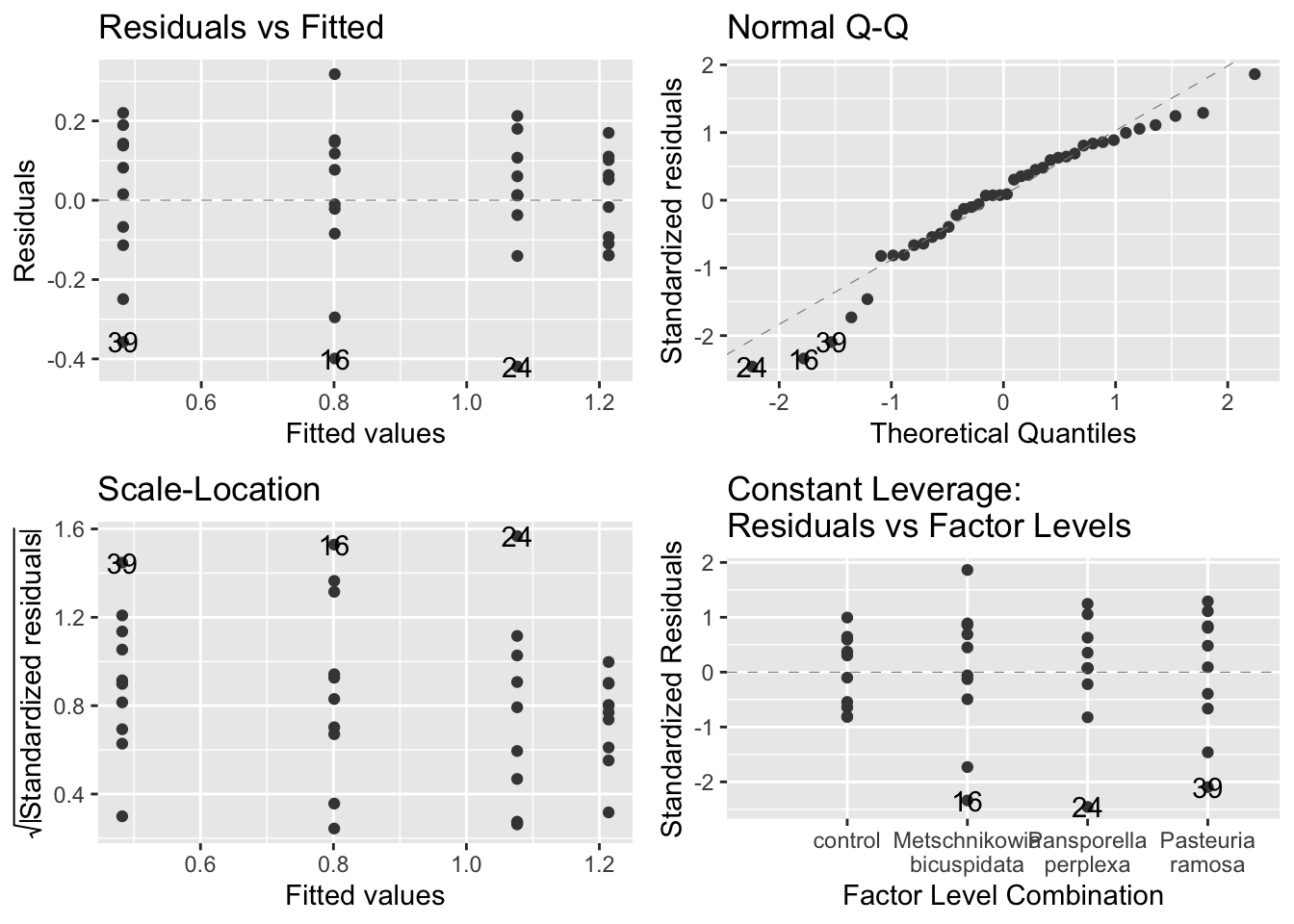• assumption-checking plots:
• The figures suggest that the assumptions are fine
• Even the upper right plot, the Q-Q plot, is within the bounds of expected variation

Produce anova table for model:

anova(model_grow)
## Analysis of Variance Table
##
## Response: growth.rate
##           Df Sum Sq Mean Sq F value    Pr(>F)
## parasite   3 3.1379 1.04597  32.325 2.571e-10 ***
## Residuals 36 1.1649 0.03236
## ---
## Signif. codes:  0 '***' 0.001 '**' 0.01 '*' 0.05 '.' 0.1 ' ' 1
• For a one-way anova, the null hypothesis is that all of the groups come from populations with (statistically) the same mean
• The F-value quantifies how likely this is to be true
• F value is the ratio between the between group variation: within group variation
• A large F value means that the between group variation is much larger
summary(model_grow)
##
## Call:
## lm(formula = growth.rate ~ parasite, data = daphnia)
##
## Residuals:
##      Min       1Q   Median       3Q      Max
## -0.41930 -0.09696  0.01408  0.12267  0.31790
##
## Coefficients:
##                                   Estimate Std. Error t value Pr(>|t|)
## (Intercept)                        1.21391    0.05688  21.340  < 2e-16 ***
## parasiteMetschnikowia bicuspidata -0.41275    0.08045  -5.131 1.01e-05 ***
## parasitePansporella perplexa      -0.13755    0.08045  -1.710   0.0959 .
## parasitePasteuria ramosa          -0.73171    0.08045  -9.096 7.34e-11 ***
## ---
## Signif. codes:  0 '***' 0.001 '**' 0.01 '*' 0.05 '.' 0.1 ' ' 1
##
## Residual standard error: 0.1799 on 36 degrees of freedom
## Multiple R-squared:  0.7293, Adjusted R-squared:  0.7067
## F-statistic: 32.33 on 3 and 36 DF,  p-value: 2.571e-10
• Output:
• table of coefficients:
• What is labelled as ‘(Intercept)’ is the control
• R tends to list things in alphabetical order
• Of the levels of the explanatory variable, ‘control’ is the first alphabetically
• In this context, we can assume that (Intercept) refers to the first level in alphabetical order - control - in this case
• Treatment contrasts report the differences between the reference level (the control in this case) and the other levels
• So -0.41275 is the difference between the control level and the parasiteMetschnikowia bicuspidata level
• In other words, the differences are the distances between the colored diamonds and the dotted black line in figure 6.1 below

Determine the means for each level:

sumDat <- daphnia %>%
group_by(parasite) %>%
summarise(meanGR = mean(growth.rate))
sumDat
## # A tibble: 4 × 2
##   parasite                  meanGR
##   <chr>                      <dbl>
## 1 control                    1.21
## 2 Metschnikowia bicuspidata  0.801
## 3 Pansporella perplexa       1.08
## 4 Pasteuria ramosa           0.482

Manually determine the difference between control and other levels:

0.8011541   -1.2139088  
##  -0.4127547
1.0763551 - 1.2139088
##  -0.1375537
0.4822030 - 1.2139088
##  -0.7317058
ggplot(daphnia, aes(x = parasite, y=growth.rate, color = parasite)) +
geom_point(size =2) +
geom_point(data = sumDat, aes( x = parasite, y = meanGR), shape = 18, size = 5) +
geom_hline(yintercept = sumDat$meanGR, lwd=1, linetype = 'dotted', colour="black") + geom_hline(yintercept = sumDat$meanGR,
lwd=1,
linetype = 'dotted',
colour="black") +
xlab("")+
theme_bw() +
coord_flip()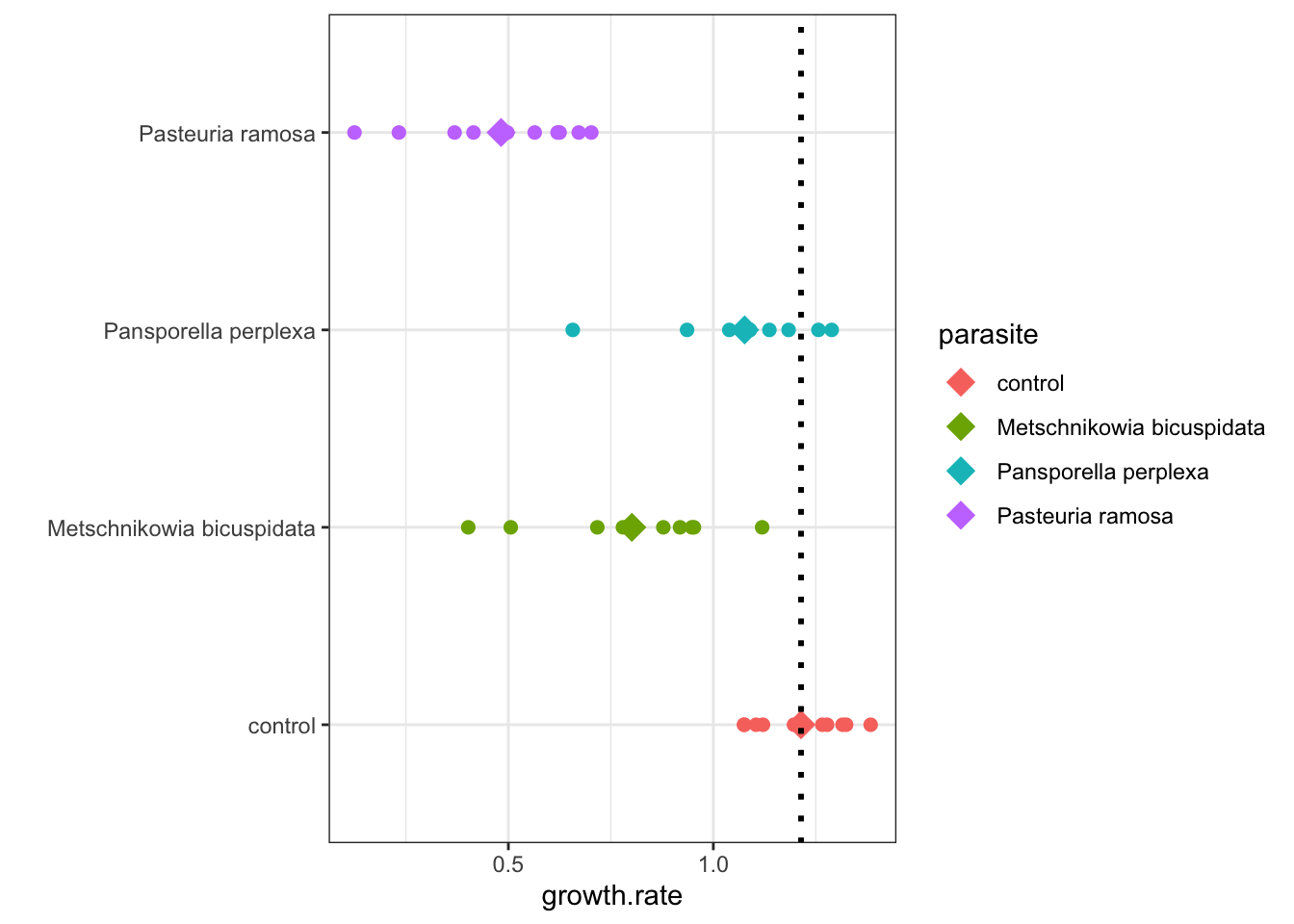Figure 5.1: Daphnia Treatment Differences